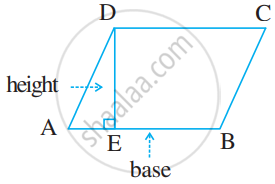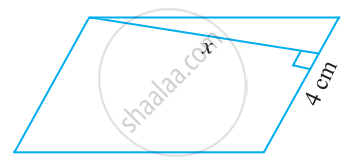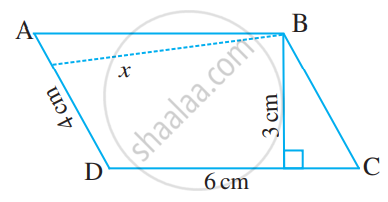# Area of a Parallelogram

#### formula

Area of parallelogram = base x height

# Area of the parallelogram:

To get the area of the parallelogram, we first draw a perpendicular line segment from one corner of the parallelogram to the opposite side. This is the height (h) of the parallelogram. The area of a parallelogram is equal to the product of its length and height.

Area of a parallelogram = base x height

You may notice that lh is also the area of a rectangle with dimensions l and h. The diagram below will explain why. If we cut out the triangle ABC and add it to the other side (triangle DEF), you will have a rectangle with dimensions l and h that has the same area as the original parallelogram.Area of parallelogram ABCD = (base x height)

• Any side of a parallelogram can be chosen as the base of the parallelogram.

• The perpendicular dropped on that side from the opposite vertex is known as height (altitude).

#### Example

Find the height ‘x’ if the area of the parallelogram is 24 cm2 and the base is 4 cm.Area of parallelogram = b × h

Therefore, 24 = 4 × x

24/4 = x

x = 6 cm

So, the height of the parallelogram is 6 cm.

#### Example

The two sides of the parallelogram ABCD are 6 cm and 4 cm. The height corresponding to the base CD is 3 cm.
Find the
(i) area of the parallelogram.
(ii) the height corresponding to the base AD.(i) Area of parallelogram = b × h
= 6 cm × 3 cm = 18 cm2

(ii) base (b) = 4 cm, height = x (say), Area = 18 cm2

Area of parallelogram = b × x

18 = 4 × x

18/4 = x

Therefore, x = 4.5 cm

Thus, the height corresponding to base AD is 4.5 cm.

If you would like to contribute notes or other learning material, please submit them using the button below.

### Shaalaa.com

Area of parallelogram [00:09:00]
S
0%